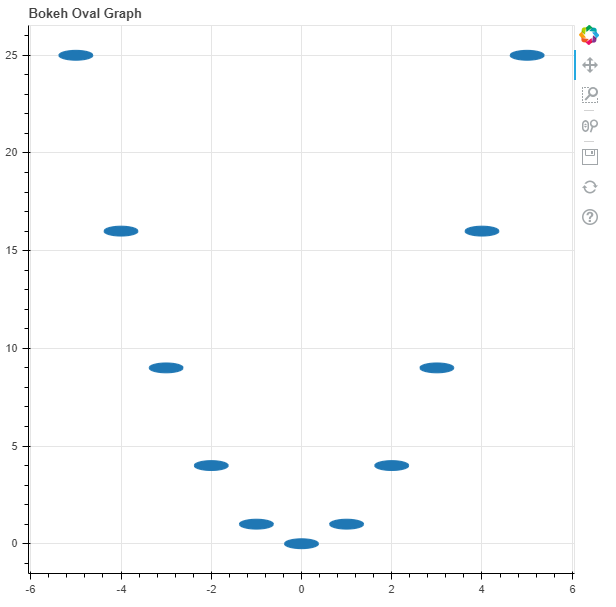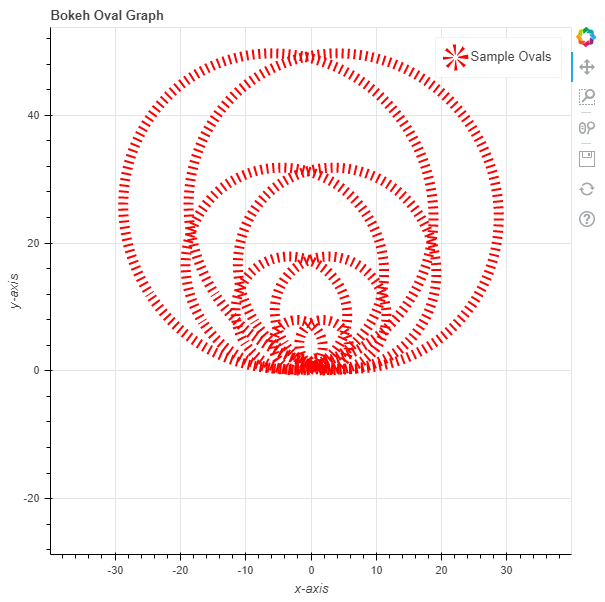Open in App
Not now

# Python Bokeh – Plotting Ovals on a Graph

• Last Updated : 10 Jul, 2020

Bokeh is a Python interactive data visualization. It renders its plots using HTML and JavaScript. It targets modern web browsers for presentation providing elegant, concise construction of novel graphics with high-performance interactivity.

Bokeh can be used to plot ovals on a graph. Plotting ovals on a graph can be done using the `oval()` method of the `plotting` module.

## plotting.figure.oval()

Syntax : oval(parameters)

Parameters :

• x : x-coordinates of the center of the oval markers
• y : y-coordinates of the center of the oval markers
• width : width of the oval markers
• height : height of the oval markers
• width_units : unit of the width of the oval markers
• height_units : unit of the height of the oval markers
• angle : angle of rotation of the oval markers, default is 0
• angle_units : unit of the angle, default is rad
• fill_alpha : fill alpha value of the oval markers
• fill_color : fill color value of the oval markers
• line_alpha : percentage value of line alpha, default is 1
• line_cap : value of line cap for the line, default is butt
• line_color : color of the line, default is black
• line_dash : value of line dash such as : solid, dashed, dotted, dotdash, dashdot [default is solid ]
• line_dash_offset : value of line dash offset, default is 0
• line_join : value of line join, default in bevel
• line_width : value of the width of the line, default is 1
• name : user-supplied name for the model
• tags : user-supplied values for the model

Other Parameters :

• alpha : sets all alpha keyword arguments at once
• color : sets all color keyword arguments at once
• legend_field : name of a column in the data source that should be used
• legend_group : name of a column in the data source that should be used
• legend_label : labels the legend entry
• muted : determines whether the glyph should be rendered as muted or not, default is False
• name : optional user-supplied name to attach to the renderer
• source : user-supplied data source
• view : view for filtering the data source
• visible : determines whether the glyph should be rendered or not, default is True
• x_range_name : name of an extra range to use for mapping x-coordinates
• y_range_name : name of an extra range to use for mapping y-coordinates
• level : specifies the render level order for this glyph

Returns : an object of class `GlyphRenderer`

Example 1 : In this example we will be using the default values for plotting the graph.

 `# importing the modules ` `from` `bokeh.plotting ``import` `figure, output_file, show ` `     `  `# file to save the model ` `output_file(``"gfg.html"``) ` `     `  `# instantiating the figure object ` `graph ``=` `figure(title ``=` `"Bokeh Oval Graph"``) ` `   `  `# the points to be plotted ` `x ``=` `[``-``5``, ``-``4``, ``-``3``, ``-``2``, ``-``1``, ``0``, ``1``, ``2``, ``3``, ``4``, ``5``] ` `y ``=` `[i ``*``*` `2` `for` `i ``in` `x] ` `  `  `# plotting the graph ` `graph.oval(x, y, ` `           ``height ``=` `0.5``, ` `           ``width ``=` `1``) ` `   `  `# displaying the model ` `show(graph) `

Output :Example 2 : In this example we will be plotting the ovals with dotted lines alongside other parameters and the size of the hexagons are in proportion to their values.

 `# importing the modules ` `from` `bokeh.plotting ``import` `figure, output_file, show ` `    `  `# file to save the model ` `output_file(``"gfg.html"``) ` `    `  `# instantiating the figure object ` `graph ``=` `figure(title ``=` `"Bokeh Oval Graph"``) ` `  `  `# name of the x-axis ` `graph.xaxis.axis_label ``=` `"x-axis"` `   `  `# name of the y-axis ` `graph.yaxis.axis_label ``=` `"y-axis"` `  `  `# the points to be plotted ` `x ``=` `[``-``5``, ``-``4``, ``-``3``, ``-``2``, ``-``1``, ``0``, ``1``, ``2``, ``3``, ``4``, ``5``] ` `y ``=` `[i ``*``*` `2` `for` `i ``in` `x] ` `  `  `# width of the ovals ` `height ``=` `[i ``*` `2` `for` `i ``in` `y] ` ` `  `# angle of the ovals ` `width ``=` `[i ``*` `2.5` `for` `i ``in` `y] ` `  `  `# angle of the ovals ` `angle ``=` `10` ` `  `# fill color value ` `fill_color ``=` `None` ` `  `# color of the line ` `line_color ``=` `"red"` `   `  `# type of line ` `line_dash ``=` `"dotted"` `   `  `# offset of line dash ` `line_dash_offset ``=` `1` `  `  `# width of the dashes ` `line_width ``=` `10` `  `  `# name of the legend ` `legend_label ``=` `"Sample Ovals"` `   `  `# plotting the graph ` `graph.oval(x, y, ` `          ``height ``=` `height, ` `          ``width ``=` `width, ` `          ``angle ``=` `angle, ` `          ``fill_color ``=` `fill_color, ` `          ``line_color ``=` `line_color, ` `          ``line_dash ``=` `line_dash, ` `          ``line_dash_offset ``=` `line_dash_offset, ` `          ``line_width ``=` `line_width, ` `          ``legend_label ``=` `legend_label) ` `  `  `    `  `# displaying the model ` `show(graph) `

Output :My Personal Notes arrow_drop_up
Related Articles